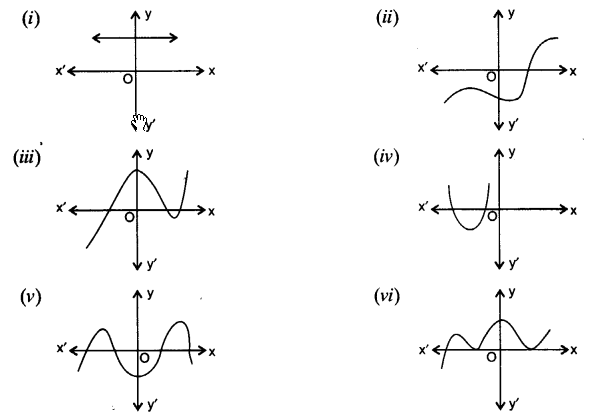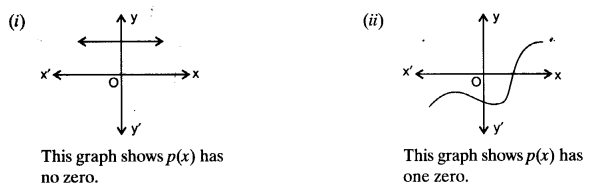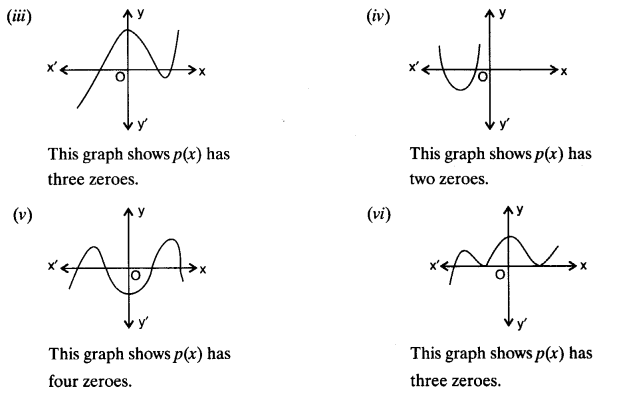# NCERT Solutions for Class 10 Maths Chapter 2 Polynomials Ex 2.1

NCERT Solutions for Class 10 Maths Chapter 2 Polynomials Ex 2.1 are part of NCERT Solutions for Class 10 Maths. Here we have given NCERT Solutions for Class 10 Maths Chapter 2 Polynomials Ex 2.1. https://mcqquestions.guru/ncert-solutions-for-class-10-maths-chapter-2-1/

 Board CBSE Textbook NCERT Class Class 10 Subject Maths Chapter Chapter 2 Chapter Name Polynomials Exercise Ex 2.1 Number of Questions Solved 1 Category NCERT Solutions

LearnInsta.com designed free Maths NCERT Solutions Class 10 to score more marks in your CBSE examination.

## NCERT Solutions for Class 10 Maths Chapter 2 Polynomials

Exercise 2.1

Question 1.
The graphs of y = p(x) are given in figure below, for some polynomials p(x). Find the number of zeroes of p(x), in each case.Solution:
The number of zeroes of p(x) in each graph given; areWe hope the NCERT Solutions for Class 10 Maths Chapter 2 Polynomials Ex 2.1 help you. If you have any query regarding NCERT Solutions for Class 10 Maths Chapter 2 Polynomials Ex 2.1, drop a comment below and we will get back to you at the earliest.